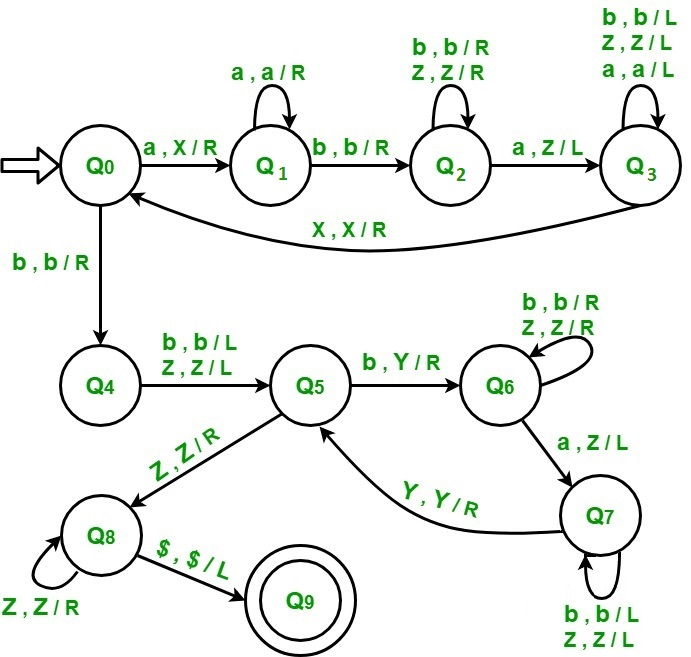# Construct Turing machine for L = {an bm a(n+m) | n,m≥1}

• Difficulty Level : Basic
• Last Updated : 31 May, 2018

L = {an bm a(n+m) | n,m≥1} represents a kind of language where we use only 2 character, i.e., a and b. The first part of language can be any number of “a” (at least 1). The second part be any number of “b” (at least 1). The third part of language is a number of “a” whose count is sum of count of a’s in first part of string and count of b’s in second part of string . Any such string which falls in this category will be accepted by this language. The beginning and end of string is marked by \$ sign.

Examples:

Attention reader! Don’t stop learning now.  Practice GATE exam well before the actual exam with the subject-wise and overall quizzes available in GATE Test Series Course.

Learn all GATE CS concepts with Free Live Classes on our youtube channel.

```Input  : a a b b b a a a a a  // n=2, m=3
Output : Accepted

Input  : a a b a a a a       // n=2, m=1
Output : Not accepted
```

Approach used –

1. Convert “a” in first part into “X” and then move right ignoring all intermediate symbols. When “a” is encountered just after “b” then convert it into “Z” and move left and stop at the position just next to “X”. Repeat the above procedure.

2. When all a’s in first part have been converted then apply the same process on second part. Convert “b” into “Y” and “a” into “Z” in the third part.

When entire first and second part has been converted and if third part is also converted then string will be accepted else not.

Steps –

Step-0: Convert “a” into “X”, move right and go to state 1. If symbol is “b”, ignore it, move right and go to state-4.

Step-1: If symbol is “a”, ignore it and move right, remain on same state. If symbol is “b”, ignore it, move right and go to state-2.

Step-2: If symbol is “Z”, ignore it and move right, remain on same state. If symbol is “b”, ignore it and move right, remain on same state and if symbol is “a”, convert it into “Z”, move left and go to state-3.

Step-3: If symbol is “Z”, ignore it and move left, remain on same state. If symbol is “b”, ignore it and move left, remain on same state. If symbol is “a”, ignore it and move left, remain on same state, and if symbol is “X”, ignore it and move right, go to state-0.

Step-4: If symbol is “b”, ignore it and move left, go to state 5, and if symbol is “Z”, ignore it and move left, go to state-5.

Step-5: Convert “b” into “Y”, move right and go to state 6, and if symbol is “Z”, ignore it and move right, go to state-8.

Step-6: If symbol is “Z”, ignore it and move right, remain on same state. If symbol is “b”, ignore it and move right, remain on same state, and if symbol is “a”, convert it into “Z”, move left and go to state-7.

Step-7: If symbol is “Z”, ignore it and move left, remain on same state. If symbol is “b”, ignore it and move left, remain on same state, and if symbol is “Y”, ignore it and move right, go to state-5.

Step-8: If symbol is “Z”, ignore it and move right, remain on same state, and if symbol is “\$”, ignore it and move left, go to state-9.

Step-9: String ACCEPTEDMy Personal Notes arrow_drop_up